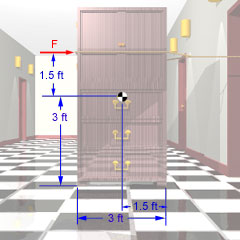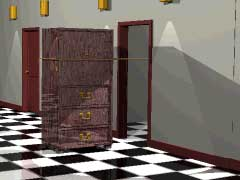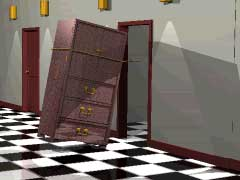Ch 6. Rigid Body Force and Acceleration Multimedia Engineering Dynamics Moment of Inertia Translational Motion Fixed Point Rotation GeneralPlane Motion
 Chapter - Particle - 1. General Motion 2. Force & Accel. 3. Energy 4. Momentum - Rigid Body - 5. General Motion 6. Force & Accel. 7. Energy 8. Momentum 9. 3-D Motion 10. Vibrations Appendix Basic Math Units Basic Equations Sections Search eBooks Dynamics Fluids Math Mechanics Statics Thermodynamics Author(s): Kurt Gramoll ©Kurt GramollDYANMICS - CASE STUDY IntroductionProblem Diagram A college student attempts to move her wardrobe into her dorm room by dragging it across the floor with a rope. If she is not careful, she may tip it over! What is known: The wardrobe weighs 200 lb. The center of gravity is located in the exact center of the wardrobe, which is 6 ft high by 3 ft wide. The rope creates a horizontal force on the wardrobe 1.5 ft above the center of gravity. The coefficient of friction (static and kinetic) between the legs of the wardrobe and the floor is μ = 0.30.Sliding WardrobeTipping Wardrobe Question If the student pulls on the rope with a 75 lb force, what are the normal forces on the legs? What is the acceleration of the wardrobe? What is the largest force that can be applied before the wardrobe will begin to tip over? Approach Use Newton's Second Law to determine the linear motion. Since there is no rotational motion, an equation involving the equilibrium of moments may be used about the center of gravity if an additional equation is required.

Practice Homework and Test problems now available in the 'Eng Dynamics' mobile app
Includes over 400 problems with complete detailed solutions.
Available now at the Google Play Store and Apple App Store.